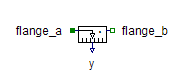Multiphase Signal Current - MapleSim Help

Multiphase Signal Current

Multiphase sine current sourceDescription The Multiphase Signal Current (or Signal Current) component contains $m$ single-phase signal current source models, each connected between corresponding phases of ${\mathrm{plug}}_{p}$ and ${\mathrm{plug}}_{n}$, with the control from the corresponding signal in the $i$ vector input.Connections

 Name Description Modelica ID ${\mathrm{plug}}_{p}$ Positive $m$-phase plug plug_p ${\mathrm{plug}}_{n}$ Negative $m$-phase plug plug_n $i$ Real vector input; current in $A$ iParameters

 Name Default Units Description Modelica ID $m$ $3$ Number of phases mModelica Standard Library The component described in this topic is from the Modelica Standard Library. To view the original documentation, which includes author and copyright information, click here.